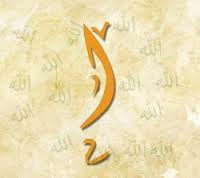### The 17 Suras Ending with the Letter Alef

#### الجمعة، 8 مايو 2015 - 2:42In the Quran 17 chapters end of (A) Alef The first chapter is number 17 with verses 111 111 = Alef 5 of chapters have prime numbers 5 of verses have 5 prime numbers 3 of total C+V have prime number 5+5+3 = 13 All of that primes Sum chapters = 976 Sum verses = 825 976+825 = 1801 prime 976 – 825 = 151 prime Digit sum of 1801 = 10 Digit sum of 151 = 7 10 + 7 = 17 prime 10 – 7 = 3 prime Digit sum of 976 = 22 Digit sum of 825 = 15 22 + 15 = 37 prime 22 – 15 = 7 prime C V C+V 17 111 128 18 110 128 19 98 117 25 77 102 33 73 106 35 45 80 48 29 77 53 62 115 65 12 77 71 28 99 72 28 100 76 31 107 78 40 118 79 46 125 86 17 103 91 15 106 110 3 113 976 825 1801 Total 22 15 Digit Sum 1801 151 -/+ 10 7 Digit Sum

 الأكثر قراءة
الموقع الرسمي حم © 2015, كل الحقوق محفوظة |